Question

# What is the angular momentum of a hydrogen atom in the following states (give your answer...

What is the angular momentum of a hydrogen atom in the following states (give your answer as a multiple of :

a. 3p state b. 4f state.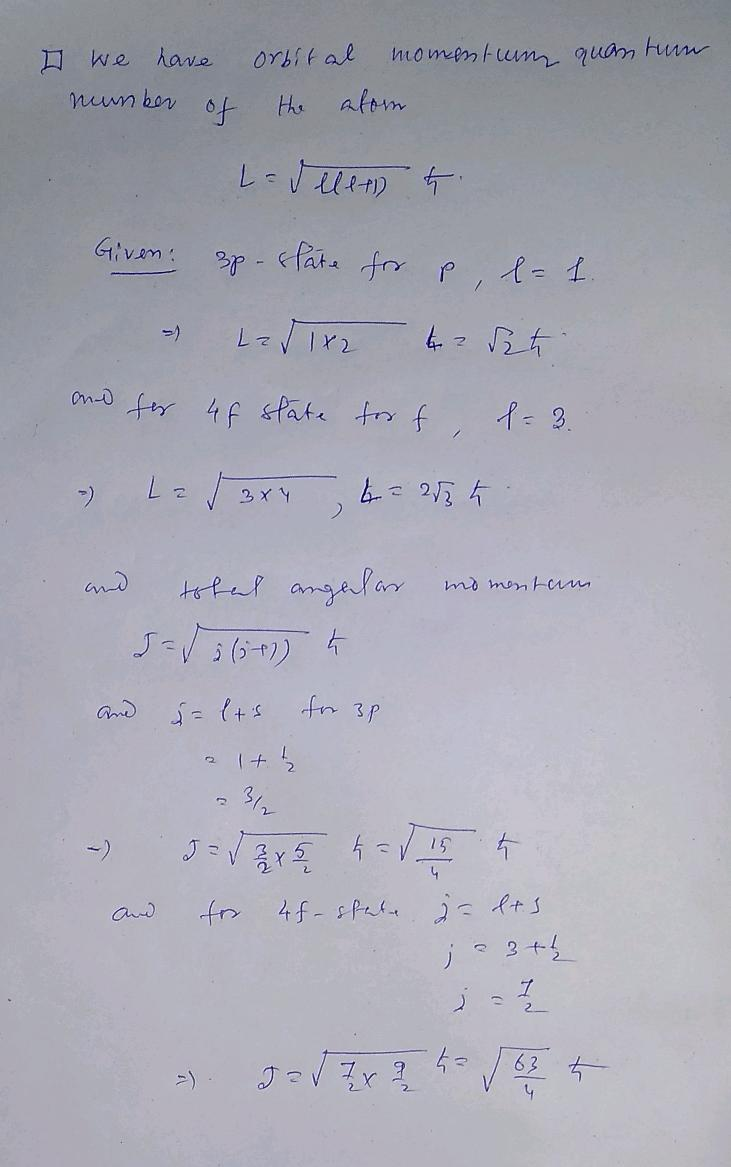#### Earn Coins

Coins can be redeemed for fabulous gifts.

Similar Homework Help Questions
• ### A hydrogen atom undergoes a transition from the 3p state to the 4d state. 1- Write...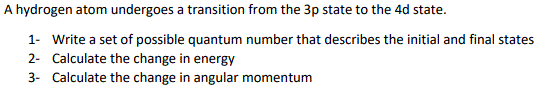A hydrogen atom undergoes a transition from the 3p state to the 4d state. 1- Write a set of possible quantum number that describes the initial and final states 2- Calculate the change in energy 3- Calculate the change in angular momentum A hydrogen atom undergoes a transition from the 3p state to the 4d state. 1. Write a set of possible quantum number that describes the initial and final states 2- Calculate the change in energy 3- Calculate the...

• ### . A hydrogen atom has angular momentum V12h. Determine the lowest energy state that the atom...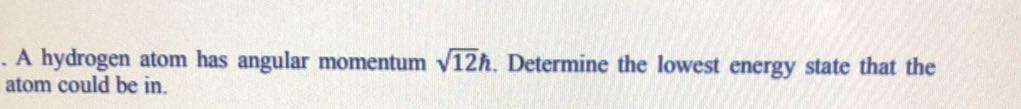. A hydrogen atom has angular momentum V12h. Determine the lowest energy state that the atom could be in.

• ### Total angular momentum An electron in a hydrogen atom has orbital angular momentum quantum number =...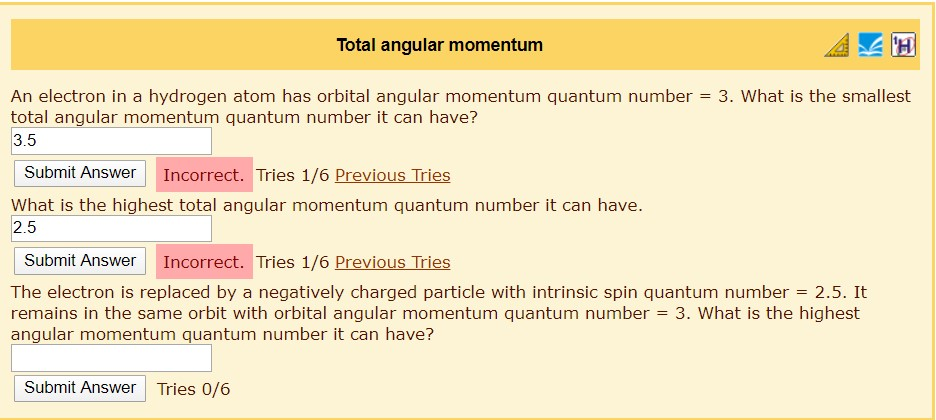Total angular momentum An electron in a hydrogen atom has orbital angular momentum quantum number = 3. What is the smallest total angular momentum quantum number it can have? 3.5 Submit Answer Incorrect. Tries 1/6 Previous Tries What is the highest total angular momentum quantum number it can have. 2.5 Submit Answer Incorrect. Tries 1/6 Previous Tries The electron is replaced by a negatively charged particle with intrinsic spin quantum number = 2.5. It remains in the same orbit with...

• ### What is the maximum possible angular momentum L (as a multiple of ?) of a hydrogen...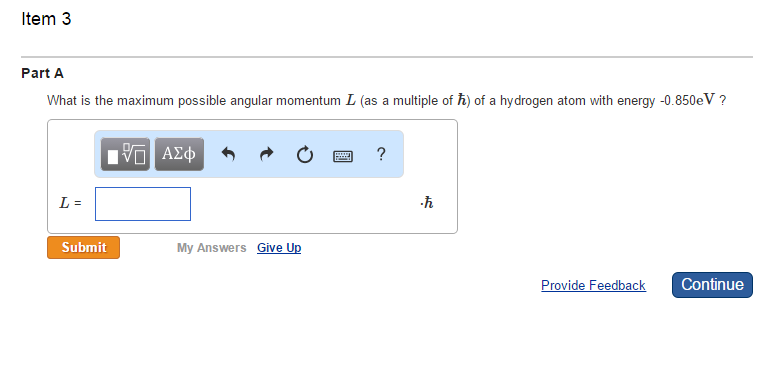What is the maximum possible angular momentum L (as a multiple of ?) of a hydrogen atom with energy -0.850eV ?

• ### Calculate the magnitude of the maximum orbital angular momentum Lmax for an electron in a hydrogen...

Calculate the magnitude of the maximum orbital angular momentum Lmax for an electron in a hydrogen atom for states with a principal quantum number of 4. Calculate the magnitude of the maximum orbital angular momentum Lmax for an electron in a hydrogen atom for states with a principal quantum number of 24. Calculate the magnitude of the maximum orbital angular momentum Lmax for an electron in a hydrogen atom for states with a principal quantum number of 179. THANK YOU

• ### An electron in a Hydrogen atom is in a state with orbital angular momentum 2 (a)...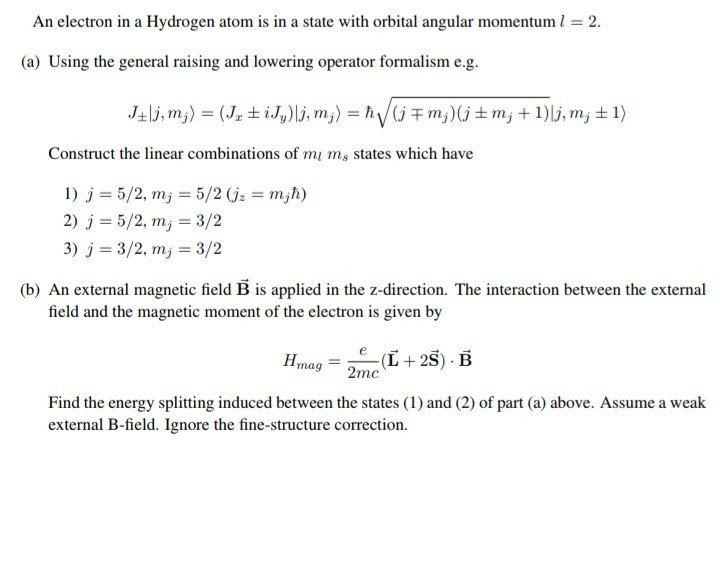An electron in a Hydrogen atom is in a state with orbital angular momentum 2 (a) Using the general raising and lowering operator formalism e.g Construct the linear combinations of mi ms states which have 2) j 5/2,my 3/2 3) j-3/2, m,-3/2 (b) An external magnetic field B is applied in the z-direction. The interaction between the external field and the magnetic moment of the electron is given by Hmag_ 2mc Find the energy splitting induced between the states (1)...

• ### Part A Calculate the magnitude of the maximum orbital angular momentum Lmax for an electron in...

Part A Calculate the magnitude of the maximum orbital angular momentum Lmax for an electron in a hydrogen atom for states with a principal quantum number of 9. Express your answer in units of ℏ to three significant figures. Part B Calculate the magnitude of the maximum orbital angular momentum Lmax for an electron in a hydrogen atom for states with a principal quantum number of 32. Express your answer in units of ℏ to three significant figures. Part C...

• ### 9. Consider the following list of orbitals in the hydrogen atom: 3p 3d     4f     5s 5p...

9. Consider the following list of orbitals in the hydrogen atom: 3p 3d     4f     5s 5p Which of these orbitals is highest in energy in the hydrogen atom? 3p is highest in energy 3d is highest in energy 4f is highest in energy 5p is highest in energy 5s and 5p are equal in energy, and are both highest in energy 10. Neodymium, atomic number 60, is a metal that is used in making very strong permanent magnets. Consider the...

• ### Electron of the hydrogen atom is at 1 = 4 orbit. a. What is the magnitude...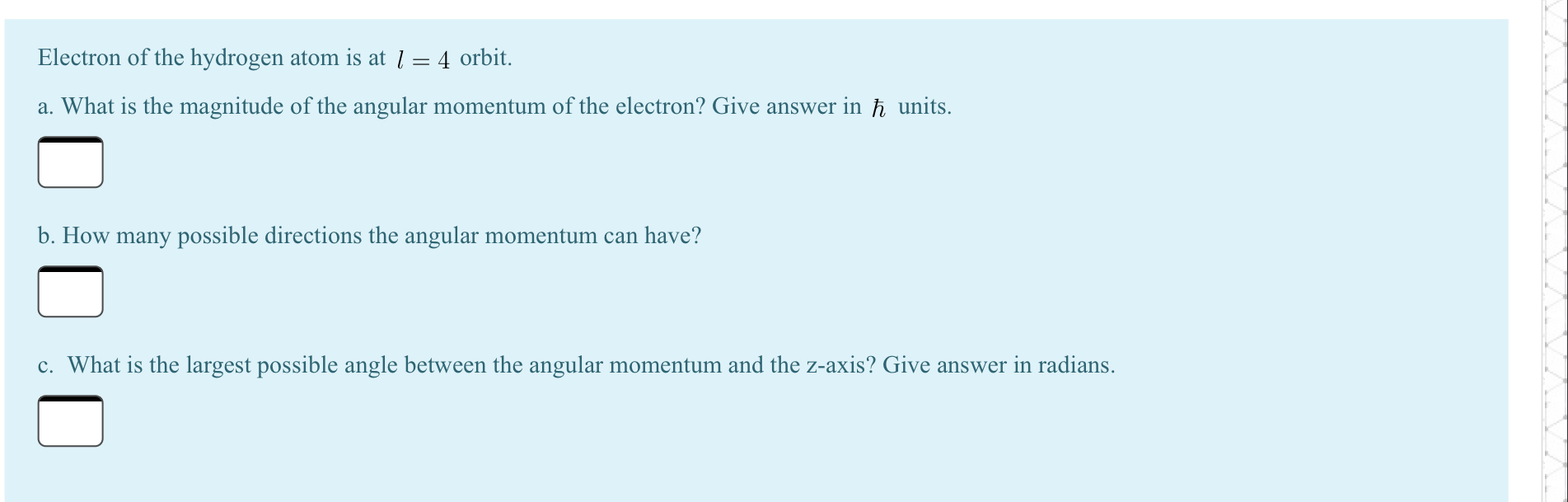Electron of the hydrogen atom is at 1 = 4 orbit. a. What is the magnitude of the angular momentum of the electron? Give answer in ħ units. b. How many possible directions the angular momentum can have? c. What is the largest possible angle between the angular momentum and the z-axis? Give answer in radians.

• ### 2C. In quantum mechanics what is the maximum angular momentum of an electron in the n...

2C. In quantum mechanics what is the maximum angular momentum of an electron in the n = 4 quantum state of the hydrogen atom? 2D. In quantum mechanics what is the maximum value for the z-component of the angular momentum of the electron in the n = 3 quantum state of the hydrogen atom?